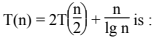Open in App
Not now

# UGC-NET | UGC NET CS 2017 Jan – III | Question 31

• Last Updated : 15 Dec, 2021

The asymptotic upper bound solution of the recurrence relation given by(A) O(n2)
(B) O(n lg n)
(C) O(n lg lg n)
(D) O(lg lg n)

Explanation:
We will apply master theorem in this question:
a = 2, b = 2 and k =1 and p = -1.
we will compare a and bk
ie

```2 = 21 and we have p = -1
Then T(n) = Θ(nlog22log log n)
T(n) = Θ(nlog log n)
and Θ(n log log n) >= O(n log log n)```

So, option (C) is correct.

Quiz of this Question

My Personal Notes arrow_drop_up